Elliott Sound Products Beginners' Guide to Transformers - Part 4

Transformers - The Basics (Part 4)

Copyright © 2017 - Rod Elliott (ESP)
Page Created November 2017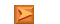Articles IndexMain Index

Contents - Part 4
Introduction

This section is something of a compendium of other articles in the series, and the reader may wonder why I keep adding material. Surely enough has been written already? Enough probably has been written, but the level of confusion about transformers in general has not abated. I see questions that need not be asked, followed by answers that are clearly wrong. Occasionally, I also see facts put forward, but unfortunately that's not often enough.

There is still a great deal of debate and confusion about power factor (there are several articles dedicated to this topic), and people insist (quite incorrectly) that a rectifier followed by a filter capacitor represents a capacitive (reactive) load. This is utter nonsense - the load is non-linear and has exactly zero reactive component. To read more on this topic, see Power Factor - The Reality and Power Factor Correction. Reactive power factor is not a consideration with small transformers, and especially so for those used in power supplies for amplifiers or preamps. Non-linear power factor is an issue that needs to be covered (again).

It's disappointing that so many people seem to know so little about transformers. They tend to be seen as 'simple' machines, and (apparently) not worthy of detailed analysis by users. The fact is that transformers are vastly more complex than assumed, and designers face a constant challenge to maximise performance and reduce prices. Toroidal transformers used to be both uncommon and very expensive, but today they are often the same price (or cheaper) than more traditional types. There can be little doubt that some of the cheapest have been 'reverse engineered' by low cost manufacturers, using a properly designed unit as the inspiration. Few people have the detailed knowledge and (expensive) commercial software that's used to perfect a design from scratch.

This short section discusses inductance, impedance and winding resistance. As noted in the other transformer articles, for normal mains transformers inductance is not part of the specification, and can be considered 'incidental'. It has to exist to limit the no load current to a reasonably sensible value, but the greatest proportion of the magnetising current is due to partial saturation. Most mains transformers have to be tested at a voltage well below their specified mains input voltage to be able to measure the inductance. A typical 230V transformer will need to be measured at no more than around 50V or so to obtain the actual inductance.

1 - Inductance

Having measured the primary inductance, you quickly discover that this information is useless - you can't do anything with it, and it doesn't help your understanding one iota. This is partly due to the simple fact that it changes. As the flux density within the core is varied, so too is the measured inductance, so it really is a useless parameter. Transformers are designed to obtain the voltage and current desired at the secondary, and the design process is based on the number of primary turns needed to get the desired no-load ('magnetising') current.

It's largely a balancing act. For a given core size, a higher magnetising current is the result of using fewer turns on the primary, and that improves regulation because the wire can be larger. However, if the no-load current is too high, the transformer will overheat because the core saturates, and the primary current is too high. A transformer that is never operated at no load can be designed to be far smaller than otherwise.

As noted several times (because it's important) ...

For any power transformer, the maximum flux density is obtained when the transformer is idle.

Measuring the inductance of a mains transformer is completely pointless. You will be able to measure it, but the value obtained has no meaning. The inductance can (perhaps - at a stretch) be considered a 'figure of merit', but the only thing that really matters is the total magnetising current, including the effects of partial saturation. Don't imagine for one minute that normal mains transformers don't saturate - every transformer I have ever measured will draw between 2 to 5 times the current you'd expect based on the inductance alone. Of course, at normal operating voltages the two are inseparable.

2 - Impedance

As noted in Section 2, transformer does not have a defined impedance. You can be excused for thinking otherwise, but that's because some transformers are designed for valve amplifier output stages or 'impedance matching' (for example). The impedance ratios are determined to match the anode resistance/ impedance of particular output valves, and convert that to an impedance suitable for a loudspeaker. In this role, the inductance of the primary winding is important, because it needs to be high enough to ensure good coupling between the valves and speakers at the lowest frequency of interest.

This is covered briefly in Section 1, and is examined in more detail in Section 2. While the inductance is important, it's even more important to ensure that the core remains well away from even partial saturation at the lowest frequencies. This is why good output transformers are so large and expensive. However, it's important to understand that while the transformer is designed and advertised as being (for example) 600Ω:600Ω, that doesn't mean that the transformer itself has these impedances. What it does mean is that it's designed for 'line level' isolation, and it has enough inductance to ensure that low frequency performance is maintained when the transformer is presenting a 600Ω load to external equipment.

As noted above, a transformer reflects the load on its secondary to its primary, and it's the load that determines the primary characteristics.

Impedance is not a consideration for mains transformers. Being powered from the mains, the source impedance is typically less than 1 ohm. The only time you'll need to work out impedances for a mains transformer is if you decide to create a simulation model, where you either use an 'ideal' (i.e. lossless and perfect) transformer with measured primary and secondary resistances added as part of the transformer, or you use an ideal voltage source with a single resistance that represents all winding resistances combined. This is discussed next.

3 - Winding Resistance & Regulation

Impedance is not a useful parameter for mains transformers, but the winding resistance determines the full-load losses and load regulation. You usually don't need to worry too much, but there may be situations where it becomes essential to be able to work out the loaded voltage, or the voltage with no (or light) loading. Some circuits don't need regulated supply voltages, but the actual voltage needs to be within a certain range to ensure that heat is minimised and parts aren't stressed unnecessarily. If you are using a regulator, you need to be certain that the voltage remains high enough to allow the regulator enough headroom for proper operation.

To ensure that a transformer is suitable for the task you intend, then you need to be able to determine that the regulation will be acceptable for your needs. This is especially true where the output of the rectifier is going to be regulated. You must ensure that the voltage remains high enough for the regulator to function properly, regardless of load current (which must be within the ratings for the transformer) and mains variations. The following table is useful, because it lets you see the transformer's regulation, based on the VA rating. While it would be nice if all transformers were fully specified (regulation, winding resistances, etc.) this is rarely the case.

 VA Resistance Regulation VA Resistance Regulation 4 1100 30% 225 8 8% 6 700 25% 300 4.7 6% 10 400 20% 500 2.3 4% 15 250 18% 625 1.6 4% 20 180 15% 800 1.4 4% 30 140 15% 1000 1.1 4% 50 60 13% 1500 0.8 4% 80 34 12% 2000 0.6 4% 120 22 10% 3000 0.4 4% 160 12 8%

This table was shown earlier, and has the info you need to judge the approximate VA rating for a transformer, or get a rough idea of the regulation you can expect (assuming that these details are not supplied). The table is only a rough guide - it is not intended to be treated as gospel, because there are many conflicting requirements that can influence the winding resistance and/or regulation in either direction. As noted, the figures are for nominal 230V transformers - if you are in a 120V country, the resistance values shown should be divided by 4 (close enough), but regulation will be similar for a given transformer rating.

A worked example is necessary here, because it's counter-intuitive that a 120V transformer should have a resistance that's 1/4 of that for 230V. It's all about the winding loss, and fewer turns on a 120V transformer mean that the wire can be thicker. Assume a primary current of 100mA at 230V (23VA for the sake of convenience). The winding resistance will be about 180 ohms (as per the table above) so primary loss will be I² * R = 0.1² * 180 = 1.8W. At 120V, the winding resistance will be 45 ohms, and primary current will be 200mA (close enough). Primary loss is therefore 0.2² * 45 = 1.8W. This is as it must be - transformers maintain the same efficiency regardless of the primary voltage if properly made. Ideally, the secondary losses will be equal to the primary losses, but this isn't always possible with small transformers (less than 50VA).

The figures for regulation in the table are based on a resistive load, and it is always worse when the output feeds a rectifier and filter capacitor. To determine the no load output voltage, you can use the figure for regulation as a guide, aided by a little maths. Let's assume you have a 20VA transformer, intended for 230v operation. The regulation will be around 15%, meaning that the no load voltage must be about 15% higher than the loaded voltage. If the rated secondary voltage is 15V, the no load voltage must be ...

 Vo = Vl / ( 1 - 0.15 ) where Vo is no-load output voltage, Vl is loaded output voltage and 0.15 is the percentage expressed as a decimal fraction Vo = 15 / 0.85 = 17.65V AC

From this, you can calculate the transformer's equivalent resistance (or impedance), knowing that a 20VA transformer can supply 1.33A at 15V into a resistive load. If 1.33A causes a voltage drop of 2.65V, the effective series resistance (or impedance) is 1.99 ohms (the secondary resistance can often be ignored, but not with very small transformers which may have a significant secondary resistance). This means that the transformer can be simulated as an ideal AC voltage source with a 2 ohm series resistor. It also behaves in the same way in 'real life', and it's the series resistance that causes the voltage to fall under load. This is worse with a rectifier and capacitor filter than for a resistive load, because the peak current is much higher. Note that all calculations are approximate. Attempting great accuracy is pointless, because the mains voltage is also variable (up to ±10%, sometimes more).

You can also use a different technique to determine the approximate primary series resistance. However, this method only gives the equivalent resistance for the primary, and doesn't include the secondary. If you have a meter that will accurately measure low resistances, the calculated primary equivalent resistance and measured secondary resistance can be added together to get the total. Using the same transformer as before (20VA, 15V secondary and 230V primary), calculate the turns ratio ...

 tr = Vp / Vs where tr is turns ratio, Vp is primary voltage and Vs is nominal secondary voltage tr = 230 / 15 = 15.33 Zr = tr² = 235 where Zr is impedance ratio, and is the square of the turns ratio

Since the primary resistance is 180 ohms (from the table), the equivalent secondary resistance is 0.765 ohm (180 / 235). Now, you must measure the secondary resistance and add them together. Assuming that the previous calculation was fairly close to reality, we can determine that the secondary resistance must be 2 - 0.765 = 1.235 ohms which can be confirmed by measurement (an accurate reading will require a low ohms meter). As already noted, all of these calculations are approximate, but are close enough for most real-world applications. If you happen to be working with mains distribution systems, then you need to go a lot deeper than I've shown here!

Note that there are two definitions for voltage regulation [ 1 ]. This isn't helpful, but they exist nonetheless. In the first case, regulation is defined as 'regulation down', meaning that the regulation is defined by the fall of the open circuit voltage to some other voltage that exists when the transformer is loaded. The second case is 'regulation up', where the loaded voltage is the reference, and it increases with no load (or only a light load). The second is less intuitive, but (especially small) transformers are almost always rated for a given secondary voltage at full load, and the unloaded voltage is not specified and must be calculated as shown. The first definition can only be used where both the loaded and unloaded voltages are specified, and this is very uncommon for small trannies. Both formulae are shown below, and Vo and Vl have the same meanings as above ...

 Regulation (down) = ( Vo - Vl ) / Vo × 100 Regulation (up) = ( Vo - Vl ) / Vl × 100

Now it's time to look at an example.

4 - 'Real World' Example

I measured a transformer in my workshop, rated at 240V in (not 230V as is the 'standard' now), 12V out at 1A. The unloaded output voltage was 14.34V. Primary resistance measured 243 ohms, and secondary resistance is 1.36 ohms. This is a 12VA transformer, but has a primary resistance that indicates (from the table above) closer to 15VA. I measured the loaded output voltage using a 12Ω resistor, and the loaded output voltage was 12.22V. We also have enough info to calculate the output voltage.

First, we'll calculate the equivalent resistance of the primary. The turns ratio is 20:1 so the impedance ratio is 400:1. With a primary resistance of 243 ohms, that works out to be a secondary equivalent of 0.607Ω (243 / 400), we now add the measured secondary resistance (1.36Ω) to get the total equivalent series resistance (1.9675Ω, close enough to 2Ω). We know that 1A and 2 ohms is a voltage drop of 2V, so the loaded secondary voltage will be about 12.34V (a 'regulation down' figure of just under 14%, or about 16% if the 'regulation up' formula is used).

There is a discrepancy between the measured and calculated output voltage, but it's only 120mV, which could easily be explained by the accuracy of the voltmeter I used to measure the mains voltage. It's not significant, and can be ignored completely in the greater scheme of things (it's less than a 1% error).

It's also worthwhile to work out the losses, although this doesn't always work as well as you might hope because most small transformers are operated with partial core saturation (this is commonly referred to as 'magnetising current', but the actual magnetising current is much lower than that measured). That means the primary current will always be somewhat higher than expected, especially with no load. We'll press on regardless, using the sample I tested. The transformer is rated for 1A output at 12V, so ...

Turns ratio (Tr) = 240 / 12 = 20
Primary current (Ip) = 12VA / 240V = 50mA
Primary loss (Lp) = 50mA² × 243Ω = 607mW
Secondary loss (Ls) = 1A² × 1.36Ω = 1.36W

These are only calculated values, and the reality will be different. I measured the primary current at 56mA with no load, and 78mA at full load. The difference from the calculated value is due to partial core saturation. This means that the full-load primary loss is actually 1.48W, and not 607mW as calculated. Note that to measure the primary current, you must use a true-RMS meter, because the waveform is not sinusoidal and the distortion causes a large measurement error. Two oscilloscope traces show what's happening ...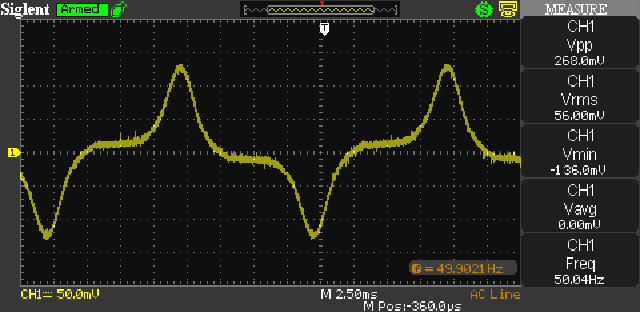Figure 1 - No-Load Primary Current Waveform

The effects of partial saturation are clearly visible, and the waveform shown is very common with all small transformers (basically anything smaller than 1kVA). The no-load loss is 762mW (commonly known as 'core loss', but this is only partially true), and this is present whenever the transformer is powered with no load connected. While the no-load loss could be reduced by using a larger core, no-one wants to pay the extra this would cost. While the end user does pay for the power consumed, this is rarely considered.

Note that the no-load loss is determined by I² × R, where I is the no-load primary current and R is the primary resistance. If you though that the loss was 240V × 56mA (13.44W), you failed to account for power factor (which cannot be determined using CosΦ, despite countless claims to the contrary). The primary current is not a sinewave, but is non-linear.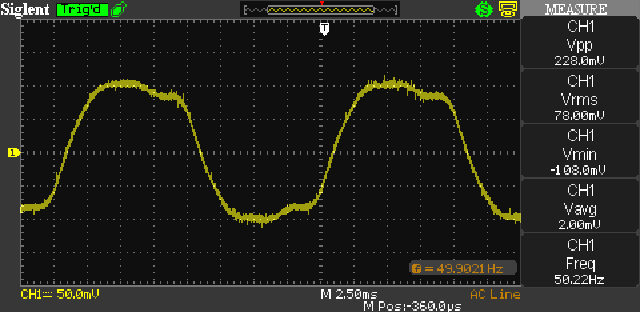Figure 2 - Full-Load Primary Current Waveform

Based on the loaded current of 78mA, you could be excused for thinking that the output current should be 1.56A, and not the 1A actually consumed by the 12 ohm load. Note that the waveform is not sinusoidal - that's because there is still some degree of core saturation (saturation current has fallen to around 28mA from the 56mA measured in Figure 1). The primary current therefore consists of 50mA of current transferred to the secondary, and 28mA that's still (partially) saturating the transformer core.

The voltage lost across the primary winding is almost 19V RMS at full load, so the original 240V applied has effectively fallen to 221V, saturation current has reduced, so therefore the flux density in the core has been reduced. The 19V lost across the primary is reflected to the secondary, reducing the available voltage by just under 1V (19V divided by the turns ratio of 20:1). The no-load voltage of 14.34V is reduced to 13.34V, with the remaining voltage (1.36V) lost across the secondary winding resistance. This should give a full-load RMS output voltage of 11.98V, but I measured 12.22V - another error!

This time, the error is caused by the fact that the incoming AC mains voltage is not sinusoidal - it's distorted by the countless non-linear loads within the distribution network. The typical mains waveform is slightly 'flat-topped', so the RMS value is a little higher than the peak value would imply (remember that the theoretical difference between peak and RMS is 1.414 (√2), but that only applies for a pure sinewave). For this reason (amongst many others), it's rather pointless trying to do exacting calculations on a transformer's performance unless you are designing it. For the end-user, you get whatever the mains and your selected transformer will give you.

So, while the measurements and calculations shown above are (hopefully) interesting, they don't provide the whole story. However, having gone through the tests and if you carry out some of your own, you will learn more about transformers, how they behave in both theory and practice, and how and why there are losses in the windings and the core. It should also be quite apparent that the statement in section 1 is indeed true ... "For any power transformer, the maximum flux density is obtained when the transformer is idle".

Many people get this completely wrong, and this is not expected to change any time soon.5 - Capacitor Input Filters

Where things become more difficult is when we load the secondary with a diode bridge and filter capacitor before the load itself. It is no longer possible to use the simple formulae we are used to, and the mere existence of the diodes and filter cap changes things in a number of unexpected ways. We need to look at the simplified equivalent circuit for a transformer, based on the calculations and measurements taken in the above section. This allows a detailed analysis of power, current and voltage at various parts of the circuit.

People generally tend to think that a 1A (AC) transformer can supply 1A DC, but this is not the case. It can be done if a switchmode regulator is used, but in most cases this is not part of the solution (and is not relevant to this discussion). The AC current into a bridge rectifier is always greater than the DC taken from the filter cap, and the ratio depends on many factors. In the following drawing, the transformer is simulated by an ideal voltage source with a series resistor that's equivalent to both the reflected primary and actual secondary resistance. This was determined to be close enough to 2 ohms.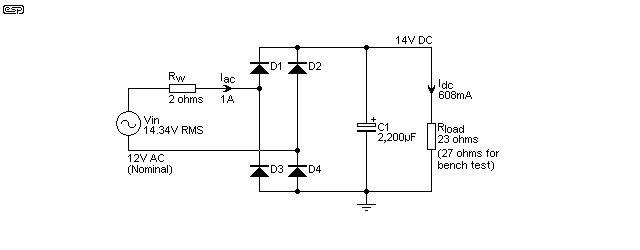Figure 3 - Transformer, Full Wave Rectifier And Capacitor Filter

The schematic shows a typical simple power supply circuit. The transformer is simulated by the voltage source and Rw (winding resistance). The AC input to the bridge rectifier is 12.78V with all values as shown. This is somewhat higher than you'd expect, but only because the waveform is distorted (which gives a higher RMS reading). The AC input voltage is 16.6V peak, but you can't use the normal crest factor (1.414) because the AC is not a sinewave. Note that the output current is 608mA for an input current of 1A RMS, meaning that the input current is nearly 1.65 times the output current. (Note that a 27 ohm resistor was used for the bench test of this circuit.)

Power in the load is 8.52W, the transformer is operating at its maximum permitted output current (1A RMS), 2W is lost in the winding resistance, and input power (from Vin) is 11.72W. The remaining 1.2W is dissipated by the four diodes (300mW each). This is remarkably close to what you will measure if you were to build an identical circuit, and shows that the theory and practice are in complete agreement. Any errors (real or imagined) are due to measurement errors and the fact that 'real' mains waveforms are pre-distorted before they even reach your circuit.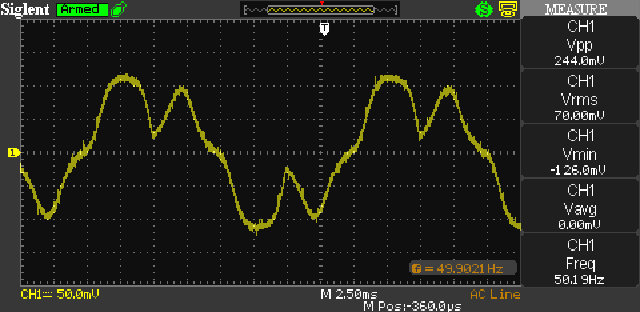Figure 4 - Primary Current Waveform, Capacitor Filter Load

The above shows the mains current waveform, and the only difference between the test circuit and that shown in Figure 3 is that the load is 27 ohms, rather than 23 ohms. It's not an especially pretty sight, and the waveform consists of saturation ('magnetising') current, and the load current with a capacitor input filter. Primary current is 70mA with a 240V mains supply. The output voltage measured 14.5V DC, very slightly higher than the simulated DC voltage (14.38V) under the same conditions. Likewise, the ripple voltage measured 520mV (RMS) vs. 473mV RMS simulated. This is to be expected, because real-world parts are never as good as a simulator will lead you to believe. However, the correlation between the measured and simulated versions is well within the tolerances one should normally expect. The secondary current with the test circuit was measured at 860mA RMS (see below) - close to the maximum for the transformer used. DC output current is 537mA into the 27 ohm load. (A simulation claimed 900mA RMS for the same conditions.)

As the transformer is made larger, winding resistance is reduced, and the peak capacitor charging current is increased. This means that the difference between AC and DC current becomes greater. The generally accepted figure is around 1.8 - i.e. assume that the AC input to the rectifier is 1.8 times as great as the DC drawn from the filter cap. However, the ratio is not a fixed 'magic' number, but depends on the winding resistance of the transformer and the size of the filter capacitor. The only way you will really find out the exact figure is to measure it (remembering to consider the mains voltage variation, thermal coefficient of resistance for copper, etc., etc.).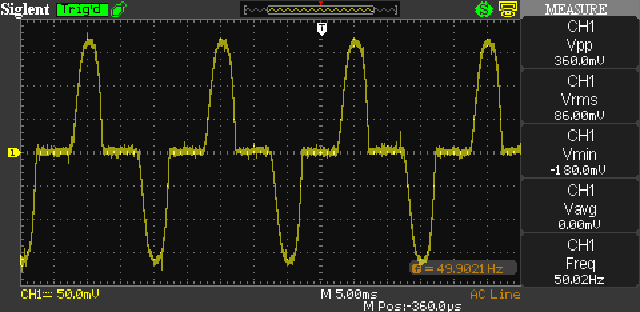Figure 5 - Secondary Current Waveform, Capacitor Filter Load

Secondary current was measured by using a 100mΩ (0.1 ohm) resistor in series with the AC to the bridge rectifier. An RMS voltage of 86mV was developed across the resistor, so current is 860mA RMS. The load was unchanged, but the DC output was reduced very slightly (about 180mV) because of the additional series resistance. You can see from the oscilloscope trace that the peak voltage dropped across the 0.1 ohm resistor is 180mV (360mV p-p).

Conclusion

The purpose of this exercise is to show that all is never as it seems when transformers are used - especially when the load is non-linear. Transformers are designed to provide their nameplate rating of volts and amps (VA), and the transformer doesn't care if the load is reactive (capacitive or inductive) or non-linear. If you exceed the VA rating, the transformer will overheat, and will eventually fail. Time to failure depends on the amount and duration of the overload. Short duration overloads usually cause no harm, provided the transformer has time to cool down again. Transformers are not rated in watts, and nor should they be. Load power is immaterial. With a purely reactive load you could easily have zero power, but the transformer will still overheat and fail if the VA rating is exceeded.

It is only by understanding what you can (and cannot) get away with that you can produce a circuit that will give a usable service life. If you don't realise that the AC secondary current can be anything from 1.6 to more than double the DC output current, then your circuit will fail due to transformer overload. Bigger transformers are actually worse than small ones in this respect. As the transformer's effective series resistance is reduced, the peak current into the capacitor is higher, and the power factor becomes worse. Power factor matters, but with the common capacitor-input filter, it is not reactive, it's non-linear.

While switching regulators give improved efficiency and a few other benefits compared to linear types, this is often not a viable solution for many projects. Switching regulators are noisy, and while the majority of the noise is outside the audio band, that doesn't always mean it can't be heard. In most hi-fi applications, hobbyists (in particular) are usually reluctant to add high frequency noise sources, because it can be very difficult to ensure that no noise is injected into the audio path.

As seen in this (and the other transformer articles), there are many things about transformers that people get (sometimes badly) wrong, and only by running simulations and/or (preferably) bench tests will you truly understand what does or does not work as expected. It's all too easy to make an assumption that turns out very badly, but people tend not to publish details of their (sometimes epic) failures. This is a shame, because you can learn a great deal more from a failure than you will from a success. This only applies if you have the will to learn of course - giving up on a problem never solves it.

Use these links for the other sections of this series.
References
1.   Electrical Machines I - Prof. Krishna Vasudevan, Prof. G. Sridhara Rao, Prof. P. Sasidhara Rao

Other reference material is in the previous 3 parts of the transformer articles. These references are not shown again here.Main IndexArticles Index

 Copyright Notice. This article, including but not limited to all text and diagrams, is the intellectual property of Rod Elliott, and is Copyright © 2017. Reproduction or re-publication by any means whatsoever, whether electronic, mechanical or electro- mechanical, is strictly prohibited under International Copyright laws. The author (Rod Elliott) grants the reader the right to use this information for personal use only, and further allows that one (1) copy may be made for reference. Commercial use is prohibited without express written authorisation from Rod Elliott.
Page created and copyright © November 2017.